# On radiation effects on hydromagnetic Newtonian liquid flow due to an exponential stretching sheet

## Abstract

The paper investigates the radiation effect on the magnetohydrodynamic Newtonian fluid flow over an exponentially stretching sheet. The effects of frictional heating and viscous dissipation on the heat transport are taken into account. The governing partial differential equations are transformed into ordinary differential equations using a suitable similarity transformation. Zero-order analytical solutions of the momentum equation and confluent hypergeometric solutions of heat and mass transport equations are obtained. The accuracy of analytical solutions is verified by numerical solutions obtained using a shooting technique that uses a Runge-Kutta-Felhberg integration scheme and a Newton-Raphson correction scheme. The effects of the radiation parameter, the magnetic parameter, Gebhart and Schmidt numbers on the momentum, heat and mass transports are discussed. The skin friction and heat and mass transfer coefficients for various physical parameters are discussed.

## 1 Introduction

The study of laminar boundary layer flow over a stretching sheet has received considerable attention in the recent past due to its immense application in industry, for example, in extrusion processes such as the polymer extrusion from a dye and wire drawing. Other engineering applications of the stretching sheet problem include polymer sheet extrusion from a dye, drawing, tinning and annealing of copper wires, glass fiber and paper production, the cooling of a metallic plate in a cooling bath and so on. There has been tremendous amount of work on the stretching sheet problem in the past several decades (see Crane , Gupta and Gupta , Grubka and Bobba , Dutta and Gupta , Siddappa and Abel , Chen and Char , Laha et al. , Chakrabarti and Gupta , Anderson et al. , Siddheshwar and Mahabaleswar , Abel and Mahesha , Abel et al.  and the references therein).

The above studies concern the linear stretching sheet problem but most of the practical situations involve a non-linear stretching sheet such as an exponential one. With this in mind, several authors have considered the velocity of the sheet to vary exponentially with the distance from the slit. Elbashbeshy  was among the first to study the exponentially stretching sheet problem. He considered a perforated sheet and examined the effect of wall mass suction on the flow and heat transfer over an exponentially stretching surface. Using a suitable similarity transformation, he transformed the momentum equation into a non-linear Riccati type equation and solved it iteratively. Ishak  studied the MHD boundary layer flow due to an exponentially stretching sheet with radiation effect. He found that the local heat transfer rate at the surface decreased with increasing values of the magnetic and radiation parameters. The flow and heat transfer from an exponentially stretching surface was considered by Magyari and Keller . They examined the heat and mass transfer characteristics and compared with the well-known results of the power-law models. Sanjayanand and Khan  studied the heat and mass transfer in a viscoelastic boundary layer flow over an exponentially stretching sheet. They found that the viscoelastic parameter enhances the thermal boundary layer thickness. The effect of viscous dissipation on the mixed convection heat transfer from an exponentially stretching surface was studied by Partha et al. . They observed a rapid growth in the non-dimensional skin friction coefficient with the mixed convection parameter. The influence of thermal radiation on the boundary layer flow due to an exponentially stretching sheet is studied by Sajid and Hayat . Khan  presented an elegant solution of the viscoelastic boundary layer flow over an exponentially stretching sheet in terms of Whittaker’s function.

The characteristics desired of the final product in an extrusion process depend on the rate of stretching and cooling. Hence, it is very important to have a controlled cooling environment where the flow over the stretching sheet can be regulated by external agencies like a magnetic field. An exponential variation of a magnetic field is used, among other applications, to determine the diamagnetic susceptibility of plasma. Steenbeck  determined the diamagnetic susceptibility of a cylindrical plasma for axial magnetic fields with various gas pressure and magnetic field strengths. Tonks  studied the effects of a magnetic field in the plasma of an arc. Pavlov  considered the magnetohydrodynamic flow of an incompressible viscous fluid over a linearly stretching surface. Sarpakaya  extended Pavlov’s work to non-Newtonian fluids. Subsequent studies by Andersson , Lawrence and Rao , Abel et al. , Cortell  concerned the magnetohydrodynamic flow of viscoelastic liquids over a stretching sheet. Radiation effects on MHD flow past an exponentially accelerated isothermal vertical plate with uniform mass diffusion in the presence of a heat source was studied by Reddy et al. . They observed that the velocity decreases with an increase in the magnetic parameter due to a resistive drag force which tends to resist the fluid flow and thus reduces the velocity. The boundary layer thickness was also found to decrease with an increase in the magnetic parameter.

In addition to a magnetic field and thermal radiation, one has to consider the viscous dissipation effects due to frictional heating between fluid layers. The effect of viscous dissipation in natural convection processes has been studied by Gebhart  and Gebhart and Mollendorf . They observed that the effect of viscous dissipation is predominant in vigorous natural convection and mixed convection processes. They also showed the existence of a similarity solution for the external flow over an infinite vertical surface with an exponential variation of surface temperature. Vajravelu and Hadjinicalaou  studied the heat transfer characteristics over a stretching surface with viscous dissipation in the presence of internal heat generation or absorption.

In this paper, we investigate the effects of various physical and fluid parameters such as the magnetic parameter, radiation parameter and viscous dissipation parameter on the flow and heat transfer characteristics of an exponentially stretching sheet. The momentum, energy and concentration equations are coupled and nonlinear. By using suitable similarity variables, these equations are converted into coupled ordinary differential equations and are solved analytically and numerically by using the Runge-Kutta-Fehlberg and Newton-Raphson schemes.

## 2 Mathematical formulation

Consider the two-dimensional magnetohydrodynamic flow of a Newtonian fluid over a stretching sheet. The origin of the system is located at the slit from which the sheet is drawn. The x-axis is taken along the continuous stretching surface and points in the direction of motion. The y-axis is perpendicular to the plate. The sheet velocity is assumed to vary as an exponential function of the distance x from the slit. The temperature and concentration far away from the fluid are assumed to be ${T}_{\mathrm{\infty }}$ and ${C}_{\mathrm{\infty }}$ respectively as shown in Figure 1. The sheet-ambient temperature and concentration differences are also assumed to be exponential functions of the distance x from the slit. A variable magnetic field of strength $B\left(x\right)$ is applied normally to the sheet. Under the usual boundary layer approximation, subject to radiation and viscous dissipation effects, the equations governing the momentum, heat and mass transports can be written as(1)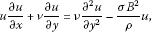(2)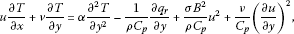(3)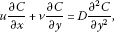(4)

where u, v are the velocity components in the x, y directions respectively, ν is the kinematic viscosity, ρ is the density, σ is the electrical conductivity of the fluid, T is the temperature, C is the concentration, $\alpha =k/\rho {C}_{p}$ is the thermal diffusivity, k is the thermal conductivity, ${C}_{p}$ is the specific heat at constant pressure, ${q}_{r}$ is the radiative heat flux, and D is the species diffusivity.

The boundary conditions for Equations (1)-(4) have the form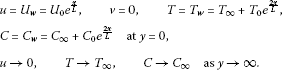(5)

Here the subscripts w, ∞ refer to the surface and ambient conditions respectively, ${T}_{0}$, ${C}_{0}$ are positive constants, ${U}_{0}$ is the characteristic velocity, and L is the characteristic length.

To facilitate a similarity solution, the magnetic field $B\left(x\right)$ is assumed to be of the form

$B\left(x\right)={B}_{0}{e}^{\frac{x}{2L}},$
(6)

where ${B}_{0}$ is a constant. It is also assumed that the fluid is weakly electrically conducting so that the induced magnetic field is negligible. Following Rosseland’s approximation, the radiative heat flux ${q}_{r}$ is modeled as

${q}_{r}=-\frac{4{\sigma }^{\ast }}{3{k}^{\ast }}\frac{\partial {T}^{4}}{\partial y},$
(7)

where ${\sigma }^{\ast }$ is the Stefan-Boltzman constant, ${k}^{\ast }$ is the mean absorption coefficient. Assuming that the temperature differences within the flow are sufficiently small such that ${T}^{4}$ may be expressed as a linear function of temperature ${T}^{4}\equiv 4{T}_{\mathrm{\infty }}^{3}T-3{T}_{\mathrm{\infty }}^{4}$, we have

$\frac{\partial {q}_{r}}{\partial y}=-\frac{16{\sigma }^{\ast }{T}_{\mathrm{\infty }}^{3}}{3{k}^{\ast }}\frac{{\partial }^{2}T}{\partial {y}^{2}}.$
(8)

Continuity Equation (1) is satisfied by introducing a stream function ψ such that

$u=\frac{\partial \psi }{\partial y},\phantom{\rule{2em}{0ex}}v=-\frac{\partial \psi }{\partial x}.$
(9)

The following similarity variables are used:

$\begin{array}{l}u={U}_{0}{e}^{\frac{x}{L}}{f}_{\eta }\left(\eta \right),\phantom{\rule{2em}{0ex}}v=-{\left(\frac{\nu {U}_{0}}{2L}\right)}^{\frac{1}{2}}{e}^{\frac{x}{2L}}\left[f\left(\eta \right)+\eta {f}_{\eta }\left(\eta \right)\right],\\ T={T}_{\mathrm{\infty }}+{T}_{0}{e}^{\frac{2x}{L}}\theta \left(\eta \right),\phantom{\rule{2em}{0ex}}C={C}_{\mathrm{\infty }}+{C}_{0}{e}^{\frac{2x}{L}}\varphi \left(\eta \right),\\ \eta ={\left(\frac{{U}_{0}}{2\nu L}\right)}^{\frac{1}{2}}y{e}^{\frac{x}{2L}},\end{array}\right\}$
(10)

where η is the similarity variable, $f\left(\eta \right)$ is the dimensionless stream function, $\theta \left(\eta \right)$ is the dimensionless temperature, and $\varphi \left(\eta \right)$ is the dimensionless concentration.

On using Equations (6), (8) and (10), Equations (2)-(5) transform into the following two-point boundary value problem: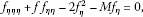(11)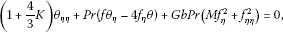(12)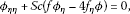(13)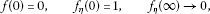(14)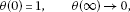(15)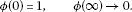(16)

The non-dimensional constants appearing in Equations (11)-(13) are the magnetic parameter M, the radiation parameter K, the Prandtl number Pr, the Gebhart number Gb, and the Schmidt number Sc respectively defined as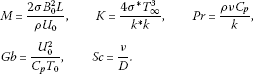## 3 Skin friction, heat and mass transfer coefficients

The parameters of engineering interest in heat and mass transport problems are the skin friction coefficient ${C}_{f}$, the local Nusselt number ${\mathit{Nu}}_{x}$, and the local Sherwood number ${\mathit{Sh}}_{x}$. These parameters respectively characterize the surface drag, wall heat and mass transfer rates.

The shearing stress at the surface of the wall ${\tau }_{w}$ is given by

${\tau }_{w}=-\mu {\left[\frac{\partial u}{\partial y}\right]}_{y=0}=-\frac{\mu {U}_{0}}{L}\sqrt{\frac{\mathit{Re}}{2}}{e}^{\frac{3x}{2L}}{f}^{″}\left(0\right),$
(17)

where μ is the coefficient of viscosity and $\mathit{Re}=\frac{L{U}_{0}}{\nu }$ is the Reynolds number. The skin friction coefficient is defined as

${C}_{f}=\frac{2{\tau }_{w}}{\rho {u}_{w}^{2}},$
(18)

and using Equation (17) in Equation (18), we obtain

$\frac{{C}_{f}\sqrt{{\mathit{Re}}_{x}/2}}{\sqrt{x/L}}=-{f}^{″}\left(0\right).$
(19)

The heat transfer rate at the surface flux at the wall is given by

${q}_{w}=-k{\left[\frac{\partial T}{\partial y}\right]}_{y=0}=\frac{-k\left({T}_{w}-{T}_{\mathrm{\infty }}\right)}{L}\sqrt{\frac{\mathit{Re}}{2}}{e}^{\frac{x}{2L}}{\theta }^{\prime }\left(0\right),$
(20)

where k is thermal conductivity of the fluid. The Nusselt number is defined as

${\mathit{Nu}}_{x}=\frac{x}{k}\frac{{q}_{w}}{{T}_{w}-{T}_{\mathrm{\infty }}}.$
(21)

Using Equation (20) in Equation (21), the dimensionless wall heat transfer rate is obtained as follows:

$\frac{{\mathit{Nu}}_{x}}{\sqrt{x/L}\sqrt{{\mathit{Re}}_{x}/2}}=-{\theta }^{\prime }\left(0\right).$
(22)

The mass flux at the surface of the wall is given by

${J}_{w}=-D{\left[\frac{\partial C}{\partial y}\right]}_{y=0}=\frac{-D\left({C}_{w}-{C}_{\mathrm{\infty }}\right)}{L}\sqrt{\frac{\mathit{Re}}{2}}{e}^{\frac{x}{2L}}{\varphi }^{\prime }\left(0\right),$
(23)

and the Sherwood is defined as

${\mathit{Sh}}_{x}=\frac{x}{D}\frac{{J}_{w}}{{C}_{w}-{C}_{\mathrm{\infty }}}.$
(24)

Using (23) in (24), the dimensionless wall mass transfer rate is obtained as

$\frac{{\mathit{Sh}}_{x}}{\sqrt{x/L}\sqrt{{\mathit{Re}}_{x}/2}}=-{\varphi }^{\prime }\left(0\right).$
(25)

In Equations (19), (22) and (25), ${\mathit{Re}}_{x}$ represents the local Reynolds number and it is defined as ${\mathit{Re}}_{x}=\frac{x{U}_{w}}{\nu }$.

## 4 Analytical solution

### 4.1 Solution of momentum equation

The momentum boundary layer equation is partially decoupled from the energy and species equations. Integrating Equation (11) with η once over to the interval $\left[0,\eta \right]$, we obtain

${f}_{\eta \eta }+f\phantom{\rule{0.2em}{0ex}}{f}_{\eta }=-s+{\int }_{0}^{\eta }\left[3{f}_{\eta }^{2}+M{f}_{\eta }\right]\phantom{\rule{0.2em}{0ex}}d\eta ,$
(26)

where $s=-{f}_{\eta \eta }\left(0\right)$. Letting $\eta \to \mathrm{\infty }$, we obtain

$s={\int }_{0}^{\mathrm{\infty }}\left[3{f}_{\eta }^{2}+M{f}_{\eta }\right]\phantom{\rule{0.2em}{0ex}}d\eta .$
(27)

Integrating Equation (26) once again, we obtain

${f}_{\eta }+\frac{1}{2}{f}_{\eta }^{2}=1-s\eta +{\int }_{0}^{\eta }\left[{\int }_{0}^{{\eta }_{1}}\left[3{f}_{{\eta }_{2}}^{2}+M{f}_{{\eta }_{2}}\right]\phantom{\rule{0.2em}{0ex}}d{\eta }_{2}\right]\phantom{\rule{0.2em}{0ex}}d{\eta }_{1}.$
(28)

The solution procedure of Equation (28) can be reduced to the sequential solutions of the Riccati type equation of the form

${f}_{\eta }^{\left(n\right)}+\frac{1}{2}{{f}^{\left(n\right)}}^{2}=\mathit{RHS}\left[{f}_{\eta }^{\left(n-1\right)}\right].$
(29)

This iteration algorithm has to be solved by substituting suitable zero-order approximations ${f}_{\eta }^{\left(0\right)}\left(\eta \right)$ for ${f}_{\eta }\left(\eta \right)$ into the right-hand side of Equation (28). We assume a zero-order approximation as

${f}_{\eta }^{\left(0\right)}\left(\eta \right)={e}^{-{s}_{0}\eta },$
(30)

which satisfies the condition at infinity. Integrating Equation (30) with respect to η and using the condition ${f}_{\eta }^{\left(0\right)}\left(0\right)=0$, we get

${f}_{\eta }^{\left(0\right)}\left(\eta \right)=\frac{1-{e}^{-{s}_{0}\eta }}{{s}_{0}}.$
(31)

Using the above solution in Equation (27), the approximate value of s can be obtained as

${s}_{0}=\sqrt{\frac{3}{2}+M},\phantom{\rule{2em}{0ex}}{f}_{\eta \eta }^{\left(0\right)}\left(0\right)=-{s}_{0}.$
(32)

Now substituting all the derivatives of zero-order approximation ${f}_{\eta }^{\left(0\right)}\left(\eta \right)$ into the right-hand side of Equation (28), we obtain the equation for first-order iteration ${f}_{\eta }^{\left(1\right)}$ as follows:

${f}_{\eta }^{\left(1\right)}+\frac{1}{2}{{f}^{\left(1\right)}}^{2}=1+\frac{3}{4{s}_{0}^{2}}\left[{e}^{-2{s}_{0}\eta }-1\right]+\frac{M}{{s}_{0}^{2}}\left[{e}^{-{s}_{0}\eta }-1\right].$
(33)

Further, we assume that the first-order iterate of f satisfies the boundary conditions on f as given in (14). The above non-linear Riccati type equation can be solved in terms of a confluent hypergeometric Whittaker function as discussed by Khan . However, we restrict ourselves to the zero-order solution, and similarly, to heat and mass transport equations.

### 4.2 Solution of heat transfer equation

Using the zero-order approximations of f and ${f}_{\eta }$ and further introducing a new variable

$\xi =-\frac{\mathit{Pr}}{{s}_{0}^{2}}{e}^{-{s}_{0}\eta },$
(34)

Equation (12) and the thermal boundary conditions (15) take the form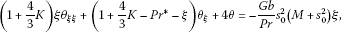(35)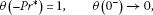(36)

where ${\mathit{Pr}}^{\ast }=\mathit{Pr}/{s}_{0}^{2}$ is the modified Prandtl number. The solution of Equation (35) is assumed in the form of

$\theta \left(\xi \right)={\theta }_{c}\left(\xi \right)+{\theta }_{p}\left(\xi \right),$

where ${\theta }_{c}\left(\xi \right)$ is the complementary solution and ${\theta }_{p}\left(\xi \right)$ is the particular solution. The complementary solution of Equation (35) is obtained in terms of confluent hypergeometric function in the following form:

${\theta }_{c}\left(\xi \right)={C}_{0}{\xi }^{\alpha }M\left[\alpha -4,\alpha +1,\frac{-\xi }{1+\frac{4}{3}K}\right],$
(37)

where

$M\left[a,b,z\right]=\sum _{r=0}^{\mathrm{\infty }}\frac{a\left(a+1\right)\cdots \left(a+r-1\right)}{b\left(b+1\right)\cdots \left(b+r-1\right)}\frac{z}{r!}$

is Kummer’s function (see Abramowitz and Stegun ) and

$\alpha =\frac{{\mathit{Pr}}^{\ast }}{1+\frac{4}{3}K}.$

The particular solution is obtained as

${\theta }_{p}\left(\xi \right)={a}_{0}{\xi }^{2}+{a}_{1}{\xi }^{3}+{a}_{2}{\xi }^{4},$
(38)

where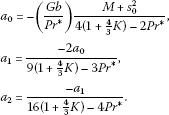Now, the complete solution can be written as

$\theta \left(\xi \right)={\theta }_{c}\left(\xi \right)+{\theta }_{p}\left(\xi \right).$
(39)

Making use of the boundary conditions (36) and rewriting the solution in terms of the variable η, we get

$\begin{array}{rcl}\theta \left(\eta \right)& =& {C}_{1}\frac{{e}^{-{s}_{0}\eta \alpha }M\left[\alpha -4,\alpha +1,-\alpha {e}^{-{s}_{0}\eta }\right]}{M\left[\alpha -4,\alpha +1,-\alpha \right]}+{a}_{0}{{\mathit{Pr}}^{\ast }}^{2}{e}^{-2{s}_{0}\eta }\\ -{a}_{1}{{\mathit{Pr}}^{\ast }}^{3}{e}^{-3{s}_{0}\eta }+{a}_{2}{{\mathit{Pr}}^{\ast }}^{4}{e}^{-4{s}_{0}\eta },\end{array}$
(40)

where

${C}_{1}=1-{a}_{0}{{\mathit{Pr}}^{\ast }}^{2}+{a}_{1}{{\mathit{Pr}}^{\ast }}^{3}-{a}_{2}{{\mathit{Pr}}^{\ast }}^{4}.$

### 4.3 Solution of mass transfer equation

Using the zero-order approximation of f and ${f}_{\eta }$ and further introducing a new variable

$\zeta =-\frac{\mathit{Sc}}{{s}_{0}^{2}}{e}^{-{s}_{0}\eta },$
(41)

Equation (13) and the thermal boundary conditions in (16) take the form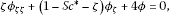(42)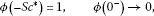(43)

where ${\mathit{Sc}}^{\ast }=\mathit{Sc}/{s}_{0}^{2}$ is the modified Schmidt number. Following the solution procedure discussed in the case of the energy equation, the solution of Equation (42) is obtained in terms of confluent hypergeometric function as

$\varphi \left(\eta \right)=\frac{{e}^{-{s}_{0}{\mathit{Sc}}^{\ast }\eta }M\left[{\mathit{Sc}}^{\ast }-4,{\mathit{Sc}}^{\ast }+1,-{\mathit{Sc}}^{\ast }{e}^{-{s}_{0}\eta }\right]}{M\left[{\mathit{Sc}}^{\ast }-4,{\mathit{Sc}}^{\ast }+1,-{\mathit{Sc}}^{\ast }\right]}.$
(44)

## 5 Solution procedure

The set of non-linear differential Equations (11)-(13) subject to the boundary conditions (14)-(16) were solved numerically using an efficient Runge-Kutta-Fehlberg method with a shooting technique, which is described in Pal and Shivakumara . The most important step in this method is to choose an appropriate finite value of $\eta \to \mathrm{\infty }$. In order to determine $\eta \to \mathrm{\infty }$ for the boundary value problem described by Equations (11)-(13), we start with initial guess values for a particular set of physical parameters to obtain ${f}^{″}\left(0\right)$, ${\theta }^{\prime }\left(0\right)$ and ${\varphi }^{\prime }\left(0\right)$. The solution procedure is repeated with another large value of $\eta \to \mathrm{\infty }$ until two successive values of ${f}^{″}\left(0\right)$, ${\theta }^{\prime }\left(0\right)$ and ${\varphi }^{\prime }\left(0\right)$ differ only by a specified significant digit. The value of η may change for a different set of physical parameters. Once the appropriate value of η is determined, the coupled boundary value problem given by Equations (11)-(13) is solved numerically using the method of superposition. In this method, third-order non-linear Equation (11), second-order Equations (12) and (13) have been reduced to five ordinary differential equations as follows:

$\begin{array}{r}{f}_{1}^{\prime }={f}_{2},\phantom{\rule{2em}{0ex}}{f}_{2}^{\prime }={f}_{3},\\ {f}_{3}^{\prime }=2{f}_{2}^{2}+M{f}_{2}-{f}_{1}{f}_{3},\\ {f}_{4}^{\prime }={f}_{5},\\ {f}_{5}^{\prime }=-\frac{\mathit{Pr}}{\left(1+\frac{4}{3}K\right)}\left[{f}_{1}{f}_{5}-4{f}_{2}{f}_{4}+\mathit{Gb}\left(M{f}_{2}^{2}+{f}_{3}^{2}\right)\right],\\ {f}_{6}^{\prime }={f}_{7},\\ {f}_{7}^{\prime }=\mathit{Sc}\left(4{f}_{2}{f}_{6}-{f}_{1}{f}_{7}\right),\end{array}$
(45)

where

${f}_{1}=f,\phantom{\rule{2em}{0ex}}{f}_{2}={f}^{\prime },\phantom{\rule{2em}{0ex}}{f}_{3}={f}^{″},\phantom{\rule{2em}{0ex}}{f}_{4}=\theta ,\phantom{\rule{2em}{0ex}}{f}_{5}={\theta }^{\prime },\phantom{\rule{2em}{0ex}}{f}_{6}=\varphi ,\phantom{\rule{2em}{0ex}}{f}_{7}={\varphi }^{\prime },$
(46)

and a prime denotes differentiation with respect to η. The boundary conditions now become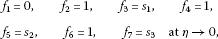(47)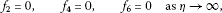(48)

where ${s}_{1}$, ${s}_{2}$ and ${s}_{3}$ are determined such that ${f}_{2}\left(\mathrm{\infty }\right)=0$, ${f}_{4}\left(\mathrm{\infty }\right)=0$ and ${f}_{6}\left(\mathrm{\infty }\right)=0$. Thus, to solve this system, we require six initial conditions. However, since we have only three initial conditions for f and two initial conditions for θ and ϕ, the conditions ${f}^{″}\left(0\right)$, ${\theta }^{\prime }\left(0\right)$, ${\varphi }^{\prime }\left(0\right)$ are to be determined by the shooting method using the initial guess values ${s}_{1}$, ${s}_{2}$ and ${s}_{3}$ until the conditions ${f}_{2}\left(\mathrm{\infty }\right)=0$, ${f}_{3}\left(\mathrm{\infty }\right)=0$ and ${f}_{5}\left(\mathrm{\infty }\right)=0$ are satisfied. In this paper, we employed the shooting technique with the Runge-Kutta-Fehlberg scheme to determine two more unknowns in order to convert the boundary value problem to an initial value problem. Once all the six initial conditions were determined, the resulting differential equations were integrated using an initial value solver. For this purpose, the fifth-order Runge-Kutta-Fehlberg integration scheme was used.

## 6 Results and discussion

Analytical and numerical solutions were obtained for the effects of radiation and viscous dissipation for the MHD flow over an exponentially stretching sheet. Similarity transformations were used to transform the governing partial differential equations of flow, heat and mass transfer into a system of non-linear ordinary differential equations. The zero-order approximate solution for the dimensionless stream function f has been obtained analytically. Solutions of the energy and species equations were obtained in terms of confluent hypergeometric functions. The accuracy of the method was established by comparing the analytical solution with the numerical solution obtained by a shooting method together with Runge-Kutta-Fehlberg and Newton-Raphson schemes. The skin friction, heat and mass transfer coefficients are tabulated in Tables 1-3. The effects of magnetic, radiation and viscous dissipation parameters on the velocity ${f}^{\prime }\left(\eta \right)$, temperature $\theta \left(\eta \right)$ and concentration $\varphi \left(\eta \right)$ profiles are shown in Figures 2-6.

Table 1 provides values of the skin friction coefficient for different values of the magnetic parameter M. Increasing values of M result in considerable opposition to the flow in the form of a Lorenz drag which enhances the values of the skin friction coefficient. Table 2 highlights the effect of the magnetic field, radiation and dissipation on the dimensionless wall temperature gradient. It is evident that all the three parameters reduce the values of the wall temperature gradient. Table 3 shows that the increase in Schmidt numbers leads to the increase in the dimensionless wall concentration gradient, while the opposite trend is observed in the case of the magnetic parameter. The results confirm a good agreement between analytical and numerical results.

The skin friction coefficients are shown in Table 4 for different values of the magnetic parameter in the absence of the physical parameters (i.e., $\mathit{Pr}=\mathit{Sc}=K=\mathit{Gb}=0$). We observe that skin friction coefficient increases with an increase in the magnetic parameter. It is interesting to note that the value of the wall skin-friction coefficient in the non-magnetic ($M=0$) and magnetic ($M=1$) cases are in good agreement with the results presented by Reddy and Reddy .

Figure 2 shows the variation of the velocity profile against the magnetic parameter. We notice that the effect of the magnetic parameter is to reduce the velocity of the fluid in the boundary layer region. This is due to an increase in the Lorentz force, similar to Darcy’s drag observed in the case of flow through a porous medium. This adverse force is responsible for slowing down the motion of the fluid in the boundary layer region. These results are similar to the results obtained by Reddy and Reddy .

The variation of the temperature distribution with the magnetic parameter is shown in Figure 3. The thermal boundary layer thickness increases with increasing values of the magnetic parameter. The opposing force introduced in the form of the Lorentz drag contributes in increasing the frictional heating between the fluid layers, and hence energy is released in the form of heat. This results in thickening of the thermal boundary layer.

The effect of the magnetic parameter on the concentration profile is shown in Figure 4. It is observed that increases in the values in M result in thickening of the species boundary layer.

The influence of the thermal radiation parameter K on temperature is shown in Figure 5. It is clear that thermal radiation enhances the temperature in the boundary layer region. Thus radiation should be kept at its minimum in order to facilitate better cooling environment. The radiation parameter K defines the relative contribution of conduction heat transfer to thermal radiation transfer.

The effect of the Gebhart number Gb on the heat transfer is shown in Figure 6. It is clear that the temperature in the boundary layer region increases with an increase in the viscous dissipation parameter. We also note that since the energy equation is partially decoupled from the momentum and species conservation equations, the parameters affecting the energy equation, namely, the Prandtl number, the radiation parameter and the Gebhart number, do not alter velocity and concentration profiles. We also observe a good agreement between the analytical and numerical solutions through Figures 2-6.

## 7 Conclusions

The problem of hydromagnetic Newtonian liquid flow due to an exponentially stretching sheet in the presence of radiation and viscous dissipation effects has been analyzed. Exact solutions were found in terms of hypergeometric functions, and a comparison of analytical and numerical results was shown. We found that the effect of the magnetic parameter is to reduce the velocity of the fluid in the boundary layer region. It was also observed that the increase in values of M results in thickening of the species boundary layer. The combined and individual effects of the magnetic parameter M, the radiation parameter K, and the viscous dissipation parameter Gb are to increase the heat transfer rates. Under some limiting conditions when the parameters Pr, Sc, K, Gb are zero, the current results agree well with available results in the literature.

## References

1. Crane LJ: Flow past a stretching plate. Z. Angew. Math. Phys. 1970, 21: 645-647. 10.1007/BF01587695

2. Gupta PS, Gupta AS: Heat and mass transfer on a stretching sheet with suction or blowing. Can. J. Chem. Eng. 1977, 55: 744-746. 10.1002/cjce.5450550619

3. Grubka LG, Bobba KM: Heat transfer characteristics of a continuous stretching surface with variable temperature. J. Heat Transf. 1985, 107: 248-250. 10.1115/1.3247387

4. Dutta BK, Gupta AS: Cooling of a stretching sheet in a various flow. Ind. Eng. Chem. Res. 1987, 26: 333-336. 10.1021/ie00062a025

5. Siddappa B, Abel MS: Non-Newtonian flow past a stretching plate. Z. Angew. Math. Phys. 1985, 36: 47-54. 10.1007/BF00949032

6. Chen CK, Char MI: Heat transfer of a continuous stretching surface with suction or blowing. J. Math. Anal. Appl. 1988, 135: 568-580. 10.1016/0022-247X(88)90172-2

7. Laha MK, Gupta PS, Gupta AS: Heat transfer characteristics of the flow of an incompressible viscous fluid over a stretching sheet. Wärme-Stoffübertrag. 1989, 24: 151-153.

8. Chakarabarti A, Gupta AS: Hydromagentic flow and heat transfer over a stretching sheet. Q. Appl. Math. 1979, 37: 73-78.

9. Andersson HI, Bech KH, Dandapat BS: Magnetohydrodynamic flow of a power-law fluid over a stretching sheet. Int. J. Non-Linear Mech. 1992, 27: 929-936. 10.1016/0020-7462(92)90045-9

10. Siddheshwar PG, Mahabaleswar US: Effects of radiation and heat source on MHD flow of a viscoelastic liquid and heat transfer over a stretching sheet. Int. J. Non-Linear Mech. 2005, 40: 807-820. 10.1016/j.ijnonlinmec.2004.04.006

11. Abel MS, Mahesha N: Heat transfer in MHD viscoelastic fluid flow over a stretching sheet with variable thermal conductivity, non-uniform heat source and radiation. Appl. Math. Model. 2008, 32: 1965-1983. 10.1016/j.apm.2007.06.038

12. Abel MS, Siddheshwar PG, Mahesha N: Effects of thermal buoyancy and variable thermal conductivity on the MHD flow and heat transfer in a power-law fluid past a vertical stretching sheet in the presence of a non-uniform heat source. Int. J. Non-Linear Mech. 2009, 44: 1-12. 10.1016/j.ijnonlinmec.2008.08.002

13. Elbashbeshy EMA: Heat transfer over an exponentially stretching continuous surface with suction. Arch. Mech. 2001, 53(6):643-651.

14. Ishak A: MHD boundary layer flow due to an exponentially stretching sheet with radiation effect. Sains Malays. 2011, 40(4):391-395.

15. Magyari E, Keller B: Heat and mass transfer in the boundary layers on an exponentially stretching continuous surface. J. Phys. D, Appl. Phys. 1999, 32: 577-585. 10.1088/0022-3727/32/5/012

16. Sanjayanand E, Khan SK: On heat and mass transfer in a viscoelastic boundary layer flow over an exponentially stretching sheet. Int. J. Therm. Sci. 2006, 45: 819-828. 10.1016/j.ijthermalsci.2005.11.002

17. Partha MK, Murthy PVSN, Rajasekhar GP: Effect of viscous dissipation on the mixed convection heat transfer from an exponentially stretching surface. Heat Mass Transf. 2005, 41: 360-366. 10.1007/s00231-004-0552-2

18. Sajid M, Hayat T: Influence of thermal radiation on the boundary layer flow due to an exponentially stretching sheet. Int. Commun. Heat Mass Transf. 2008, 35: 347-356. 10.1016/j.icheatmasstransfer.2007.08.006

19. Khan SK: Boundary layer viscoelastic fluid flow over an exponentially stretching sheet. Int. J. Appl. Mech. Eng. 2006, 11(2):321-335.

20. Steenbeck M: Wissen. Veroff. Siemens 1936., 15(2): Article ID 1

21. Tonks L: Theory of magnetic effects in the plasma of an arc. Phys. Rev. 1939, 56: 360-373. 10.1103/PhysRev.56.360

22. Pavlov KB: Magnetohydromagnetic flow of an incompressible viscous fluid caused by deformation of a surface. Magn. Gidrodin. 1974, 4: 146-147.

23. Sarpakaya T: Flow of non-Newtonian fluids in a magnetic field. AIChE J. 1961, 7: 324-328. 10.1002/aic.690070231

24. Andersson HI: MHD flow of a viscoelastic fluid past a stretching surface. Acta Mech. 1992, 95: 227-230. 10.1007/BF01170814

25. Lawrence PS, Rao BN: The non-uniqueness of the MHD flow of a viscoelastic fluid past a stretching sheet. Acta Mech. 1995, 112: 223-228. 10.1007/BF01177490

26. Abel MS, Joshi A, Sonth RM: Heat transfer in MHD viscoelastic fluid flow over a stretching surface. Z. Angew. Math. Mech. 2001, 81: 691-698.

27. Cortell R: Flow and heat transfer of an electrically conducing fluid of second grade over a stretching sheet subject to suction and to a transverse magnetic field. Int. J. Heat Mass Transf. 2006, 49: 1851-1856. 10.1016/j.ijheatmasstransfer.2005.11.013

28. Reddy PBA, Reddy NB, Suneetha S: Radiation effects on MHD flow past an exponentially accelerated isothermal vertical plate with uniform mass diffusion in the presence of heat source. J. Appl. Fluid Mech. 2012, 5(3):119-126.

29. Raptis A: Radiation and viscoelastic flow. Int. Commun. Heat Mass Transf. 1999, 26(6):889-895. 10.1016/S0735-1933(99)00077-9

30. Raptis A, Perdikis C: Viscoelastic flow by the presence of radiation. Z. Angew. Math. Mech. 1998, 78: 277-279. 10.1002/(SICI)1521-4001(199804)78:4<277::AID-ZAMM277>3.0.CO;2-F

31. Bidin B, Nazar R: Numerical solution of the boundary layer flow over an exponentially stretching sheet with thermal radiation. Eur. J. Sci. Res. 2009, 33(4):710-717.

32. Elbashbeshy EMA, Dimian MF: Effect of radiation on the flow and heat transfer over a wedge with variable viscosity. Appl. Math. Comput. 2002, 132: 445-454. 10.1016/S0096-3003(01)00205-3

33. Reddy PBA, Reddy NB: Thermal radiation effects on hydromagnetic flow due to an exponentially stretching sheet. Int. J. Appl. Math. Comput. 2011, 3(4):300-306.

34. Raptis A, Perdikis C, Takhar HS: Effect of thermal radiation on MHD flow. Int. J. Heat Mass Transf. 2004, 153: 645-649.

35. Hayat T, Abbas Z, Sajid M, Asghar S: The influence of thermal radiation on MHD flow of a second grade fluid. Int. J. Heat Mass Transf. 2007, 50: 931-941. 10.1016/j.ijheatmasstransfer.2006.08.014

36. Gebhart B: Effect of viscous dissipation in natural convection. J. Fluid Mech. 1962, 14: 225-232. 10.1017/S0022112062001196

37. Gebhart B, Mollendorf J: Viscous dissipation in external natural convection flows. J. Fluid Mech. 1969, 38: 97-107. 10.1017/S0022112069000061

38. Vajravelu K, Hadjinicolaou A: Heat transfer in a viscous fluid over a stretching sheet with viscous dissipation and internal heat generation. Int. Commun. Heat Mass Transf. 1993, 20: 417-430. 10.1016/0735-1933(93)90026-R

39. Abramowitz M, Stegun LA National Bureau of Standards, AMS 55. Handbook of Mathematical Functions 1972.

40. Pal D, Shivakumara IS: Mixed convection heat transfer from a vertical plate embedded in a sparsely packed porous medium. Int. J. Appl. Mech. Eng. 2006, 11(4):929-939.

## Acknowledgements

The authors are grateful to the National Research Foundation (NRF) and the University of KwaZulu-Natal for financial support.

## Author information

Authors

### Corresponding author

Correspondence to P Sibanda.

### Competing interests

The authors declare that they have no competing interests.

### Authors’ contributions

The problem was conceived in discussions by the authors. PKK and MN carried out the analytical and numerical computations, while GM and PS participated in the design of the study and drafted the manuscript.

## Authors’ original submitted files for images

Below are the links to the authors’ original submitted files for images.

## Rights and permissions

Reprints and Permissions

Kameswaran, P., Narayana, M., Sibanda, P. et al. On radiation effects on hydromagnetic Newtonian liquid flow due to an exponential stretching sheet. Bound Value Probl 2012, 105 (2012). https://doi.org/10.1186/1687-2770-2012-105

• Accepted:

• Published:

• DOI: https://doi.org/10.1186/1687-2770-2012-105

### Keywords

• Heat Transfer
• Viscous Dissipation
• Magnetic Parameter
• Boundary Layer Flow
• Skin Friction Coefficient